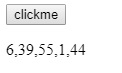# How to find the maximum value of an array in JavaScript?

we can find the Maximum value of an array using Math.max() and sort() functions.

## 1) Math.max()

Math.max() function usually takes all the elements in an array and scrutinize each and every value to get the maximum value.It's methodology is discussed in the below example.

Live Demo

### Example

<html>
<body>
<script>
function maximum(value) {
if (toString.call(value) !== "[object Array]")
return false;
return Math.max.apply(null, value);
}
document.write(maximum([6,39,55,1,44]));
</script>
</body>
</html>

### Output

55.

## 2) sort()

Using sort and compare functions we can arrange the elements in array in descending or ascending order, later on using the length property we can find the required value.

Live Demo

### Example

<html>
<body>
<input type ="button" onclick="myFunction()" value = "clickme">
<p id="max"></p>
<script>
var numbers = [6,39,55,1,44];
document.getElementById("max").innerHTML = numbers;
function myFunction() {
numbers.sort(function(a, b){return a - b});
document.getElementById("max").innerHTML = numbers;
document.write(numbers[numbers.length-1]);
}
</script>
</body>
</html>

From the above program the output is displayed as the following image### Output

Later on clicking the above  click me button the maximum value is displayed  as the following

55